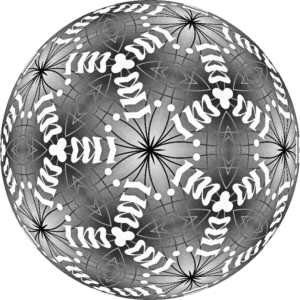Russell Lyons

Russell Lyons obtained his PhD in harmonic analysis at the University of Michigan in 1983. Within a few years, some coincidences led him to switch to probability, where he has been happily ever since. Lyons is James H. Rudy Professor of Mathematics and Adjunct Professor of Statistics at Indiana University. His primary area of research is discrete probability and its connections to other areas of mathematics, including ergodic theory, geometric group theory, analysis, geometry, and combinatorics. He is also very interested in the teaching of statistics and has done some research in statistics. Lyons was a Sloan Foundation Fellow, a Visiting Miller Research Professor, an IMS Medallion Lecturer, an Invited Speaker at the International Congress of Mathematicians, and a Simons Foundation Fellow, and gave an Hour Address at the Joint Mathematics Meetings. He is a Fellow of the American Mathematical Society.

This IMS/Bernoulli Society Schramm Lecture will be given at the IMS London meeting in June.

## Monotonicity in Continuous-Time Random Walk

Suppose that rn > 0 for all non-negative integers n. This determines a symmetric birth-and-death chain with transition rates rn from n to n+1 and from n+1 to n. That is, there are Poisson processes with rates rn such that when the Markov chain is at n, it moves to n−1 or n+1 at the next time that one of the corresponding Poisson processes has an event. Consider how long it takes the chain, starting from 0, to reach n for the first time. Is this stochastically decreasing in the set of rates? In other words, if any rate is increased, will this tend to make the time to reach n decrease? The fact that transitions occur faster suggests that the answer is yes, but the subtlety is that transitions are more likely in each direction where the rate is increased, not only in the direction that increases the location. Nevertheless, the first intuition is correct: the time does decrease stochastically. This follows from a result of Karlin and McGregor (1957).

This is but one example of a kind of monotonicity in the rates for (variable-speed) continuous-time random walk on graphs. We will survey results, counterexamples, and open questions. We will give general ideas of proofs, but avoid technicalities.

Suppose that G is a graph with positive rates on its edges, used for Poisson processes: When a walker is at a vertex, it moves to a neighbor at the time of the next event that occurs for the corresponding incident edges. The transition probability of being back at the starting vertex at time t is well known to be decreasing in t. Now, increasing t by a factor of c is equivalent to fixing t and increasing all rates by a factor of c. But what if we fix t and increase only one rate? Then this return probability need not be decreasing. However, Benjamini and Schramm (2005) proved that when G is finite, if we average the return probabilities over all starting vertices, then we do get a smaller number.

Both this result and that above on birth-and-death chains depend on a spectral comparison for Laplacian matrices. For return probabilities, there have been many extensions, including to infinite graphs. Suppose that Γ is a group generated by some finite subset, S. Let G be the corresponding Cayley graph, meaning that the vertices of G are the elements of Γ and the edges are {x,xs} for x Γ and s S. Let rs > 0 for s S. If the rate on every edge of the form {x,xs} is rs, then the return probability at time t is the same for all x Γ. Thus, when Γ is finite, there is no need to average. One of the extensions alluded to is that we do have monotonicity for all Cayley graphs, without averaging, even for infinite Cayley graphs. In many cases, we also have monotonicity for the expected return probability on a Cayley graph when the edge rates form a random field whose law is invariant under left group multiplication, and we are comparing two random fields where one has edge rates at least those of the other. But whether this same phenomenon holds for all Cayley graphs is an open question due to Fontes and Mathieu (2006), even on regular trees.

Most of our talk will be devoted to other types of monotonicity on Cayley graphs. On infinite graphs, we may ask about the limiting linear rate of escape, i.e., the limit of the distance divided by the time. Does this increase when the rates are increased? On finite graphs, we may ask about the convergence to the stationary (uniform) distribution. Does this happen faster when the rates are increased? It turns out that both questions have surprising answers. The figure [below], based on the IMS logo, has a symmetry group where monotonicity occurs in all expected ways. This is new work of the speaker with Graham White.